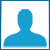330 photos   6617 visits

=)))))))))))))))))))))))))))))))))))))))))).
Yeah. <3 We`re @TheEvilTeam. (:
Haha, die. =)).
Yes. I`m hungry. Problems? =))))).
ilysm Jeydon. <3
You are moooooooooreeee than A-M-A-Z-I-N-G. <3
You`re the BOSS. B-)

- Mozzby. (: <33 -